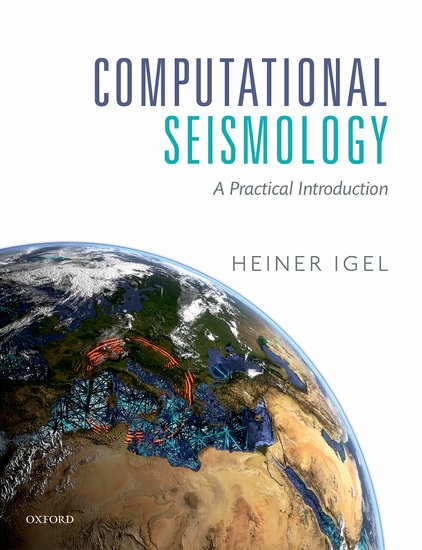Computational Seismology
Finite Differences - Advection Equation in 1DThis notebook is part of the supplementary material to Computational Seismology: A Practical Introduction, Oxford University Press, 2016.

##### Authors:¶

This exercise illustrates two types of finite difference schemes and their stability when solving the one-dimensional advection equation.

### Exercise¶

The source-free advection equation is given by

$$\partial_t u(x, t) = v \partial_x u(x, t)$$

where $u(x,t = 0)$ could be a displacement waveform at $t = 0$ (an initial condition) that is advected with velocity $v$. Replace the partial derivatives by finite-differences. Which approach do you expect to work best? Turn it into a programming exercise and write a simple finite-difference code and play around with different schemes (centered vs. non-centered finite differences). What do you observe?

#### Imports¶

The matplotlib.use("nbagg") line makes sure plots are done in the notebook at not in an external window.

In [ ]:
# This is a configuration step for the exercise. Please run it before the simulation code!
import numpy as np
import matplotlib
# Show Plot in The Notebook
matplotlib.use("nbagg")
import matplotlib.pyplot as plt


#### Setup¶

This block initializes all necessary variables, arrays, and sets the initial conditions.

In [ ]:
# Setup
nx = 1250          # Number of grid points.
v = 5500.          # Acoustic velocity in m/s.
x_max = 10000      # Length of the domain in m.
eps = 0.5          # CFL
tmax = 1.0         # Simulation time in s
isnap = 2          # Plot the solution each isnap timesteps.
sig = 100          # Sigma for the gaussian source time function
x0 = 1000          # Center point of the source time function

# Choose between a "upwind" and a "centered" finite difference scheme.
fd_type = "upwind"
#fd_type = "centered"

# Spatial setup
x = np.linspace(0, x_max, nx)
dx = x - x

# Use wave based CFL criterion, time step will be calculated from stability criterion
dt = eps * dx / v

# Simulation time
nt = int(tmax / dt)

# Initial condition in space.
sx = np.exp(-1.0 / sig ** 2.0 * (x - x0) ** 2.0);

# Initialize fields
u = sx
unew = np.zeros(nx)
du = np.zeros(nx)

# Plot the initial condition before simulating
plt.close()
plt.ioff()
plt.figure(figsize=(12, 4.5))
plt.title("Initial Conditions")
plt.grid()
plt.plot(x, u, color="black", lw=2)
plt.xlabel("x (m)")
plt.ylabel("Amplitude")
plt.show()


#### Time Loop¶

In [ ]:
# Advection Equation - Time Extrapolation

# Close plots, plot the initial condition for comparison
plt.close()
plt.figure(figsize=(12, 4.5))

lines = plt.plot(x, u, color="black", lw=1.5, label="Current State")
plt.plot(x, u, color="0.5", ls="--", lw=1, label="Initial State")

plt.xlabel("x (m)")
plt.ylabel("Amplitude")
if fd_type == "upwind":
title = "Upwind finite-difference scheme"
elif fd_type == "centered":
title = "Centered finite-difference scheme"
else:
raise ValueError("fd_type must be 'forward' or 'centered'")

plt.grid()
plt.ylim(u.min(), u.max() * 1.1)
plt.legend()
plt.ion()
plt.show()

# Here we start the actual time extrapolation, the task for you is to calculate
# the space derivative of u(x) and write it into du(x)

for i in range(nt):
du[:] = 0.0
for j in range(1, nx - 1):
if fd_type == "upwind":
du[j] = (u[j] - u[j - 1]) / dx
elif fd_type == "centered":
du[j] = (u[j + 1] - u[j - 1]) / (2.0 * dx)

# time extrapolation scheme (Euler)
unew = u - dt * v * du

# The new presence is the current future!
u = unew

# Update plot if desired.
if not i % isnap:
for l in lines:
l.remove()
del l
lines = plt.plot(x, unew, color="black", lw=1.5)
plt.title(title + ", time step: %i, time: %.2g s" % (i, i * dt))
plt.gcf().canvas.draw()

plt.ioff()
plt.show()

In [ ]: